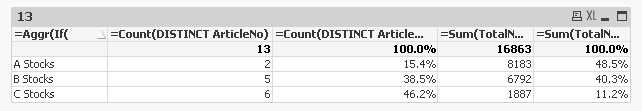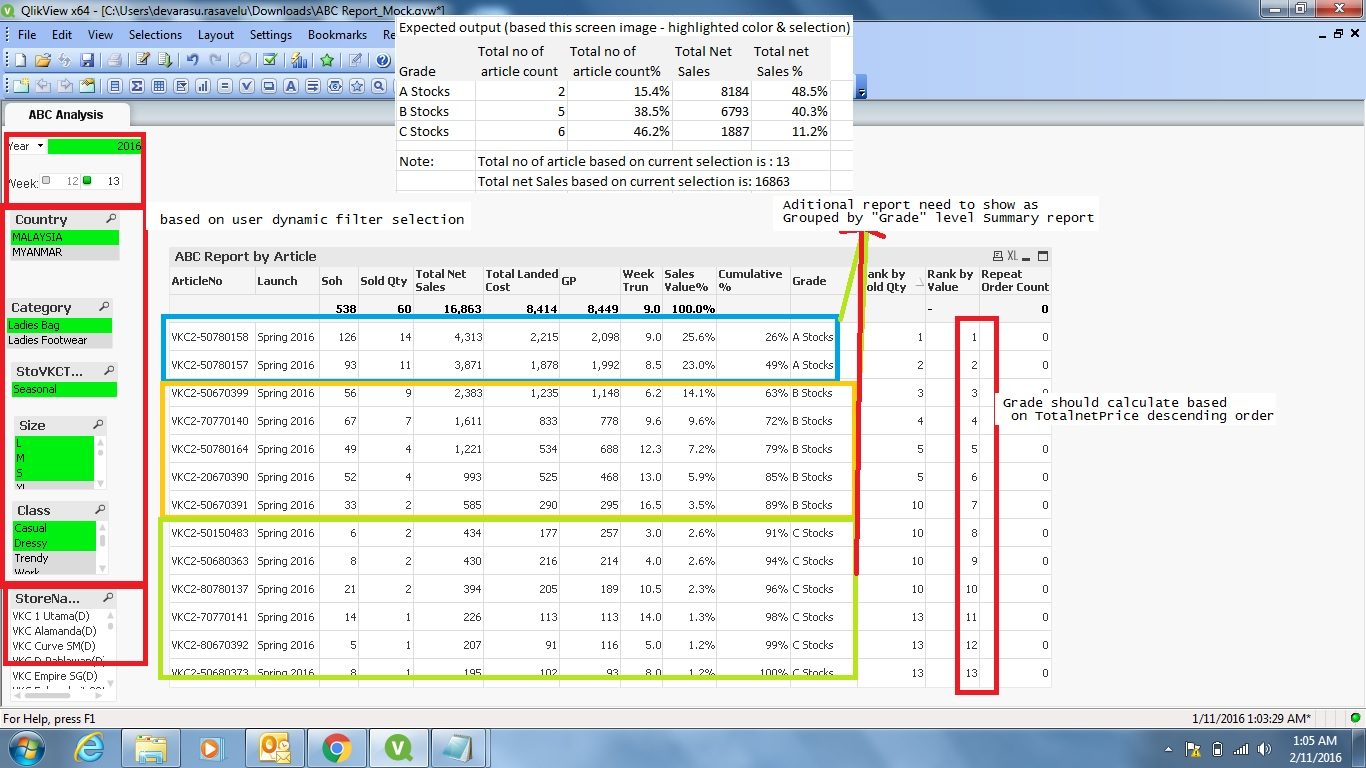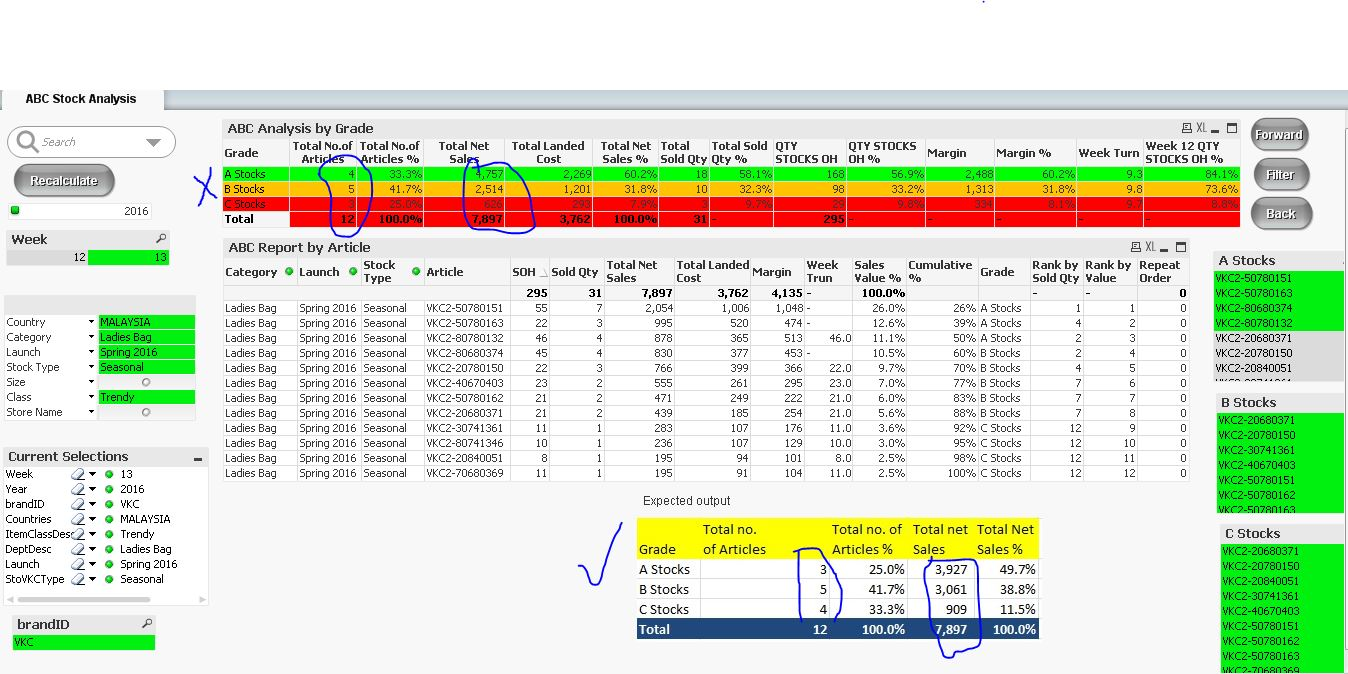# New to QlikView

Discussion board where members can get started with QlikView.

Highlighted
Honored Contributor II

## ABC Analysis in Qlikview

Hi All,

I'm trying to build ABC report for Sales analysis. having issue with calculated dimension(Grade) and based on user selection it should show bellow attached expected output screen shot, currently i'm getting wrong results (only the ABC summary report not correct result). if you have any solutions/idea's much appreciated for your help. thanks

Note: used below script for calculated dimension (Grade)

Cumulative%:

LOAD *,TotalNetPrice/TotalSales as SalVale%,

If(RangeSum(Peek('run_sum'), TotalNetPrice/TotalSales) < 0.6, 'A Stocks',

If((RangeSum(Peek('run_sum'), TotalNetPrice/TotalSales)) > 0.6 and (RangeSum(Peek('run_sum'), TotalNetPrice/TotalSales)) < 0.9 , 'B Stocks', 'C Stocks')) as Grade,

RangeSum(Peek('run_sum'), TotalNetPrice/TotalSales) as run_sum

Resident Sales

Order By TotalNetPrice desc;

FYI

Current output (refer the ABC summary report)Tags (4)
1 Solution

Accepted SolutionsMVP

## Re: ABC Analysis in Qlikview

Here is the new chart

Dimension

=Aggr(If(

rangesum(above(TOTAL (Sum({<ArticleNo>}TotalNetPrice)/Sum(TOTAL {<ArticleNo>} TotalNetPrice)),0,RowNo(Total)))<0.6,'A Stocks',if(

rangesum(above(TOTAL (Sum({<ArticleNo>}TotalNetPrice)/Sum(TOTAL {<ArticleNo>} TotalNetPrice)),0,RowNo(Total)))>0.6 and rangesum(above(TOTAL (Sum({<ArticleNo>}TotalNetPrice)/Sum(TOTAL {<ArticleNo>} TotalNetPrice)),0,RowNo(Total)))<0.9,'B Stocks',

'C Stocks'

)

), (ArticleNo, (=Sum({<ArticleNo>}TotalNetPrice), DESC)))

Expression

=Count(DISTINCT ArticleNo)

=Count(DISTINCT ArticleNo)/Count(TOTAL DISTINCT ArticleNo)

=Sum(TotalNetPrice)

=Sum(TotalNetPrice)/Sum(TOTAL TotalNetPrice)28 RepliesMVP & Luminary

## Re: ABC Analysis in Qlikview

I'm not quite sure how do you want to classify your articles but if you do it within the script it couldn't be depend on selections anymore - maybe it would be sufficient if you include more dimensions like country and category within your aggregation-load and on which you react in the following peek-load.

But I think you will rather need a calculated dimension within the gui - like described here: Calculated Dimensions and an approach like the following:

Recipe for an ABC Analysis

ABC analisys. As it should be...

Segmentation and custom dimension grouping

ABC Analysis to set and remember classification at runtime

I have adapted a chart from the first blog-posting which is based on a ranking and a second one (the left one) which based on the sales and which should be already quite near to your expected output except for the wrong sorting which will be quite difficult to create with a qlikview release prior to QV 12 - but with them it should be easy: The sortable Aggr function is finally here!

- Marcus

Honored Contributor II

## Re: ABC Analysis in Qlikview

Hi Marcus,

Thank you very much for your help, in my requirement is to calculate the grade based on calculated measure and user selected dynamic filter value.

Let's assume we have list box filter as country,Category,Week,size etc, Based on the dimension we need to calculate the Grade (Calculated dimension). would it be possible to derive below summary report by Grade

problem statement:

The problem with calculated dimension is that unable to sort the RangeSum in the descending order and it's creating incorrect Grades.

Please refer below screen image,Best Regards,

DevaMVP & Luminary

## Re: ABC Analysis in Qlikview

Have you tried my suggestion with a sortable aggr-function in QV 12 (maybe at first within a local installation)?

- Marcus

Honored Contributor II

## Re: ABC Analysis in Qlikview

Hi Marcus,

Yes, I've tried with the suggested aggregated with sorting option as below,

=Aggr(if(

rangesum(above(SUM((TotalNetPrice)/SUM(TOTAL TotalNetPrice)),0,RowNo(Total)))<0.6,'A Stocks',if(

rangesum(above(TOTAL SUM((TotalNetPrice)/SUM(TOTAL TotalNetPrice)),0,RowNo(Total)))>0.6 and

rangesum(above(TOTAL SUM((TotalNetPrice)/SUM(TOTAL TotalNetPrice)),0,RowNo(Total)))<0.9,'B Stocks',

'C Stocks'

)

),ArticleNo, (TotalNetPrice ,(NUMERIC,DESCENDING)))

But still could not yield the desired output. I have attached the current and expected output. Please share your valuable suggestion/solution for this.

Note:

I would like to have below kind functionality where i would like to sort by expression. Basically a ORDER BY.

Aggr(sum(sales),(sum(sales),(NUMERIC, ASCENDING)))

This will open up lot of possibilities. One good example is Running Total/cumulative total as Calculated Dimension.

Thank you once again.

Best Regards, DevaMVP & Luminary

## Re: ABC Analysis in Qlikview

If I look on the syntax from the new aggr-function I don't think that an expression as sorting-attribute is possible. I haven't a QV 12 available so I couldn't test anything. Perhaps there is a possibility by extending the condition for a ranking-function maybe by nesting them within another aggr-function might work. For this I would like to invite swuehl to this posting who had a lot experience with aggr-functions or he might give useful hints for a different approach.

- MarcusMVP

## Re: ABC Analysis in Qlikview

As I mentioned to you before also, I think troyansky's approach is probably the only approach (AFAIK) available, I have created a sample for you using the approach he mentioned here: So How Many Customers Make Up Most of Your Sales?

The only problem is that you are not including the boundary conditions, but Pareto Select includes it. So when you say Sum(TotalNetPrice) of less than 50%, your chart shows 2 ArticleNo, but pareto includes the anything until it crosses the 50% mark. May be someone (marcus_sommer‌, Oleg, or someone else) might be able to help you take this even forward.Oh and forgot to mention that every time you change your selections, you will need to click on Recalculate to make this work. Other method is to add the button actions as OnAnySelect action, but that could drastically slow down your dashboard. But it will definitely help you get rid of the recalculate buttonHonored Contributor II

## Re: ABC Analysis in Qlikview

Hi Sunny,

Thank you so much for the solution provided. As you said, i'm now facing with one issue. When using Pareto select, the percentage for StockA is 60. Say, 2 stocks are filtered out. The second pareto select's percentage is 90. What now happens is, this time it includes the StockA's 2 article count and also the articles which are less than 90%. When it comes to StockC, the similar thing happens. Is there anyway we can mention the percentage range instead of only the minimum value. Legends of qlikview - marcus_sommer, swuehl. Please pen down your valuable suggestion for this.

Thanks a lot in advance ! Have a wonderful weekend !

Regards, Deva

Honored Contributor II

## Re: ABC Analysis in Qlikview

Can you help me to advise on this Pareto Percentage (%) range selection issue. Thanks

need to set like below,

StockA: <=60%

StockB: >60 and <=90%

StockC: >90%Regards,

DevaMVP

## Re: ABC Analysis in Qlikview

I am out of ideas on how we can take this forward, lets get some other experts involved may be johnw‌, marcowedel